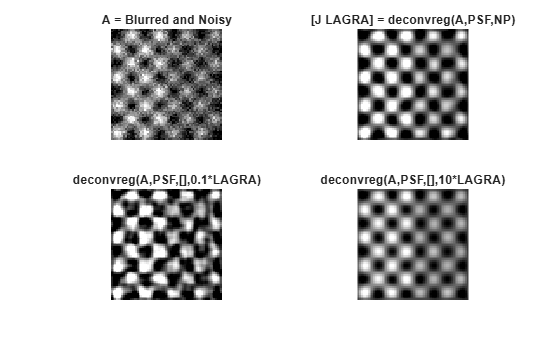deconvreg

Deblur image using regularized filter

Description

example

J = deconvreg(I,psf) deconvolves image I using the regularized filter algorithm, returning deblurred image J. The assumption is that the image I was created by convolving a true image with a point-spread function (PSF), psf, and possibly by adding noise. The algorithm is a constrained optimum in the sense of least square error between the estimated and the true images under requirement of preserving image smoothness.

J = deconvreg(I,psf,np) specifies the additive noise power, np.

J = deconvreg(I,psf,np,lrange) specifies the range, lrange, where the search for the optimal solution is performed. The algorithm finds an optimal Lagrange multiplier lagra within the lrange range.

J = deconvreg(I,psf,np,lrange,regop) constrains the deconvolution using regularization operator regop. The default regularization operator is the Laplacian operator, to retain the image smoothness.

[J,lagra] = deconvreg(___) outputs the value of the Lagrange multiplier, lagra in addition to the restored image, J.

Examples

collapse all

Create sample image.

I = checkerboard(8);

Create PSF and use it to create a blurred and noisy version of the input image.

PSF = fspecial('gaussian',7,10);
V = .01;
BlurredNoisy = imnoise(imfilter(I,PSF),'gaussian',0,V);
NOISEPOWER = V*prod(size(I));

Deblur the image.

[J LAGRA] = deconvreg(BlurredNoisy,PSF,NOISEPOWER);

Display the various versions of the image.

subplot(221); imshow(BlurredNoisy);
title('A = Blurred and Noisy');
subplot(222); imshow(J);
title('[J LAGRA] = deconvreg(A,PSF,NP)');
subplot(223); imshow(deconvreg(BlurredNoisy,PSF,[],LAGRA/10));
title('deconvreg(A,PSF,[],0.1*LAGRA)');
subplot(224); imshow(deconvreg(BlurredNoisy,PSF,[],LAGRA*10));
title('deconvreg(A,PSF,[],10*LAGRA)');Input Arguments

collapse all

Blurry image, specified as a numeric array of any dimension.

Data Types: single | double | int16 | uint8 | uint16

PSF, specified as a numeric array.

Data Types: double

Noise power, specified as a numeric scalar.

Data Types: double

Search range, specified as a numeric scalar or a 2-element numeric vector. If lrange is a scalar, then the algorithm assumes that lagra is equal to lrange. If you specify lagra, then the function ignores the np value

Data Types: double

Regularization operator, specified as a numeric array. The regop array dimensions must not exceed the dimensions of the image, I. Any nonsingleton dimensions must correspond to the nonsingleton dimensions of psf.

Data Types: double

Output Arguments

collapse all

Deblurred image, returned as a numeric array. J has the same data type as I.

Lagrange multiplier, returned as a numeric scalar.

Tips

• The output image J could exhibit ringing introduced by the discrete Fourier transform used in the algorithm. To reduce the ringing, use I = edgetaper(I,psf) before calling deconvreg.

 Gonzalez, R. C., and R. E. Woods. Digital Image Processing. Addison-Wesley Publishing Company, Inc., 1992.